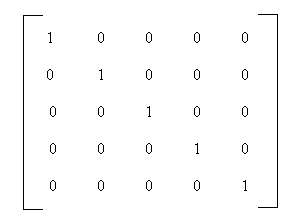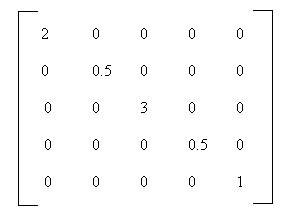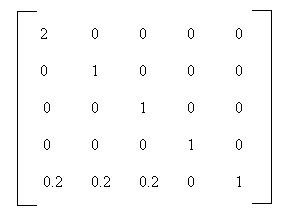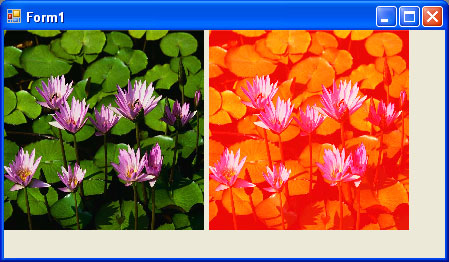# Color Transformation and the Color Matrix in GDI+

So far we have seen the transformation of graphics shapes from one state to another, but have you ever thought about transforming colors? Why would you want to transform an image's colors? Suppose you wanted to provide grayscale effects, or needed to adjust the contrast, brightness, or even "redness" of an image. For example, images retrieved from video and still cameras often need correction. In these cases, a color matrix is very useful.

As we discussed in earlier articles, the color of each pixel of a GDI+ image or bitmap is represented by a 32-bit number, of which 8 bits each are used for the red, green, blue and alpha components. Each of the four components is a number from 0 to 255. For red, green, and blue, 0 represents no intensity and 255 represents full intensity. For the alpha component, 0 represents, transparent and 255 represents fully opaque. A color vector includes four items: A, R, G, and B. The minimum values for this vector are (0, 0, 0, 0, 0), and the maximum values are (255, 255, 255, 255, 255).

GDI+ allows the use of values between 0 and 1, where 0 represents the minimum intensity and 1 the maximum intensity. These values are used in a color matrix to represent the intensity and opacity of color components. For example, the color vector with minimum values is (0, 0, 0, 0) and the color vector with maximum values is (1, 1, 1, 1).

In a color transformation we can apply a color matrix on a color vector by multiplying a 4X4 matrix. However, a 4X4 matrix supports only linear transformation such as rotation and scaling. To perform nonlinear transformations such as translation, we must use a 5X5 matrix. The element of the fifth row and the fifth column of the matrix must be 1, and all of the others entries in the five columns must be 0.

The elements of the matrix are identified according to a zero-based index. The first element of the matrix is M, and the last element is M. A 5X5 identity matrix is shown in Figure 10.21. In this matrix the elements M, M, M, and M represent the red, blue, green, and alpha factors respectively. The element M means nothing, and it must always be 1.

Now, if we want to double the intensity of the red component of a color, we simply set M equal to 2. For example, the matrix shown in Figure 10.22 doubles the intensity of the red component, decreases the intensity of the green component by half, triples the intensity of the blue component, and decreases the opacity of the color by half (making it semi-transparent).FIGURE 10.21: An identity matrixFIGURE 10.22: A matrix whose components have different intensities

In the matrix shown in Figure 10.22, we multiplied the intensity values. We can also add intensity values by using other matrix elements. For example, the matrix shown in Figure 10.23 will double the intensity of the red component and add 0.2 to each of the red, green, and blue component intensities.

The ColorMatrix Class

In this section we will discuss the ColorMatrix class. As you might guess from its name, this class defines a matrix of colors. In the preceding sections we discussed the Matrix class. The ColorMatrix class is not very different from the Matrix class. Whereas the Matrix class is used in general transformation to transform graphics shapes and images, the ColorMatrix class is specifically designed to transform colors. Before we see practical use of the color transformation, we will discuss the ColorMatrix class, its properties, and its methods.FIGURE 10.23: A color matrix with multiplication and addition

The ColorMatrix class constructor takes an array that contains the values of matrix items. The Item property of this class represents a cell of the matrix and can be used to get and set cell values. Besides the Item property, the ColorMatrix class provides 25 MatrixXY properties, which represent items of the matrix at row (x+1) and column (y+1). MatrixXY properties can be used to get and set and item's value.

LISTING 10.17: Creating a ColorMatrix object

float
[][] ptsArray =
{

new
float[] { 1, 0, 0, 0, 0},
new
float[] { 0, 1, 0, 0, 0},
new
float[] { 0, 0, 1, 0, 0},
new
float[] { 0, 0, 0, 0.5F, 0},
new
float[] { 0, 0, 0, 0, 1}};
ColorMatrix clrMatrix = new ColorMatrix(ptsArray);
if (clrMatrix.Matrix34 <= 0.5)
{
clrMatrix.Matrix34 = 0.8f;
clrMatrix.Matrix11 = 0.3f;
}
}

Section 10.8 will describe how to apply color matrices to the transformation of colors.

Matrix Operations in Image Processing

Recoloring, the process of changing image colors is a good example of color transformation. Recoloring includes changing colors, intensity, contrast, and brightness of an image. It can all be done via the ImageAttributes class and its methods.

The color matrix can be applied to an image via the SetColorMatrix method of the ImageAttributes class. The ImageAttributes object is used as a parameter when we call DrawImage.

Translating Colors

Translating colors increases or decreases color intensities by a set amount (not by multiplying them). Each color component (red, green, and blue) has 255 different levels ranging from 0 to 255. For example, assuming that the current intensity level for the red component of a color is 100. Changing its intensity level to 150 would imply translating by 50.

In a color matrix representation, the intensity varies from 0 to 1. The last row's first four elements represent the translation of red, green, blue, and alpha components of a color, as shown in Figure 10.22. Hence, adding a value to these elements will transform a color. For example, the t1, t2, t3, and t4 values in the following color matrix represent the red, green, blue, and alpha component translations, respectively:

Color Matrix = {
{1, 0, 0, 0, 0},
{0, 1, 0, 0, 0},
{0, 0, 1, 0, 0},
{0, 0, 0, 1, 0},
{t1, t2, t3, t4, 1}};

Listing 10.18 uses a ColorMatrix object to translate colors. We change the current intensity of the red component to 0.90. First we create a Graphics object using the CreateGraphics method, and we create a Bitmap object from a file. Next we create an array of ColorMatrix elements and create a ColorMatrix object from this array. Then we create an ImageAttributes object and set the color matrix using SetColorMatrix, which takes the ColorMatrix object as its first parameter. After all that, we draw two images. The first image has no effects; the second images show the result of our color matrix transformation. Finally, we dispose of the objects.

LISTING 10.18: Using ColorMatrix to translate colors

using
System;
using
System.Collections.Generic;
using
System.ComponentModel;
using
System.Data;
using
System.Drawing;
using
System.Drawing.Imaging;
using
System.Drawing.Drawing2D;
using
System.Text;
using
System.Windows.Forms;

namespace
ColorTransformationColorMatrix
{
public partial class Form1 : Form
{
public Form1()
{
InitializeComponent();
}

private void Form1_Paint(object sender, PaintEventArgs e)
{
//Create a Graphics object
Graphics g = this.CreateGraphics();
g.Clear(this.BackColor);
//Create a Bitmap object
Bitmap curBitmap = new Bitmap("c:/water.jpg");
//Color matrix elements
float[][] ptsArray =
{

new
float[] {1, 0, 0, 0, 0},
new
float[] {0, 1, 0, 0, 0},
new
float[] {0, 0, 1, 0, 0},
new
float[] {0, 0, 0, 1, 0},
new
float[] {.90f, .0F, .0f, .0f, 1},
};
//Create a ColorMatrix object
ColorMatrix clrMatrix = new ColorMatrix(ptsArray);
//Create image attributes
ImageAttributes imgAttribs = new ImageAttributes();
//Set color matrix
imgAttribs.SetColorMatrix(clrMatrix,
ColorMatrixFlag.Default,
//Draw image with no effects
g.DrawImage(curBitmap, 0, 0, 200, 200);
//Draw image with image attributes
g.DrawImage(curBitmap,
new Rectangle(205, 0, 200, 200),
0, 0, curBitmap.Width, curBitmap.Height,
GraphicsUnit.Pixel, imgAttribs);
//Dispose of objects
curBitmap.Dispose();
g.Dispose();
}
}
}

Figure 10.24 shows the output from Listing 10.18. The original image is on the left; on the right we have the results of our color translation. If you change the values of other components (red, blue, and alpha) in the last row of the color matrix, you'll see different results.FIGURE 10.24 Translating colors

Conclusion

Hope the article would have helped you in understanding Color Transformation and the Color Matrix in GDI+. Read other articles on GDI+ on the website.This book teaches .NET developers how to work with GDI+ as they develop applications that include graphics, or that interact with monitors or printers. It begins by explaining the difference between GDI and GDI+, and covering the basic concepts of graphics programming in Windows.